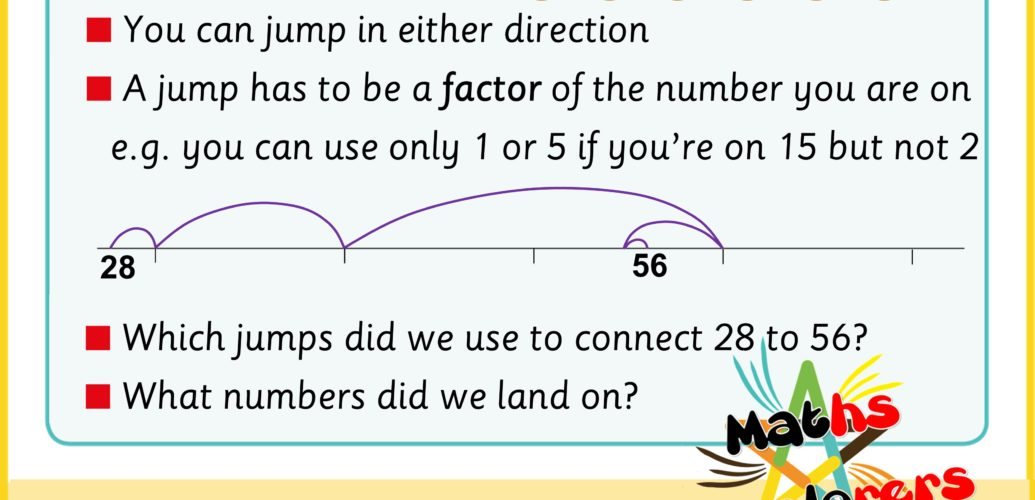# Open number lines

You are likely to be familiar with number lines that have evenly spaced numbers already printed on them. They are one of the most powerful representations in arithmetic.

They can be made to show negative numbers, multiples, fractional numbers and even extend into graph axes. They are, along with their popular alternatives, number tracks or bar models, one of the most useful visual representations of number and it is really important to become as familiar as possible with them. Most classes will have a number line displayed on the wall and we would recommend having a number line or a few number lines in the back of your maths book to refer to in order to check working.

While regular number lines are likely to be printed and used, open number lines are a way to visually represent calculations while you are doing the calculation. Being able to visually represent the abstract nature of calculation is really important if you want to develop these skills and take on more complex arithmetic. Open number lines function as a rough and easily reproducible representation of a number line. Imagine that you are working out the difference between 28 and 56, a number line might be a good way to visualise this.

Start with a line and put 28 and 56 on it. We now need to see what jumps we could do to get from 28 to 56 (or the other way round).

Probably the easiest way is to jump to 30 (a jump of 2) then to jump 20 to 50…

…and finish with a jump of 6. That means the difference between 28 and 56 is 2 + 20 + 6 which is 28.

There are lots of other ways to do it of course. One example is to jump to 60 instead and jump back 4. 2 + 30 – 4 is 28 again. Same answer, different method.

Practising this way of visualising arithmetic procedures helps you develop your ability to understand and perform mental arithmetic. It is very beneficial.

In this week’s challenge, we have introduced some constraints. They are intended to help children develop this as a way to extend their mental maths abilities. One of the constraints is the jumps that you can use. We’ve used the numbers that are most often used to estimate because they are easy to count in. You could extend the numbers to try this challenge for bigger numbers by including 100, 200 and 500 for example.

The other constraint is that you can only use jumps which are factors of the number you’re on. This helps children think about times tables and makes it more of a challenge.